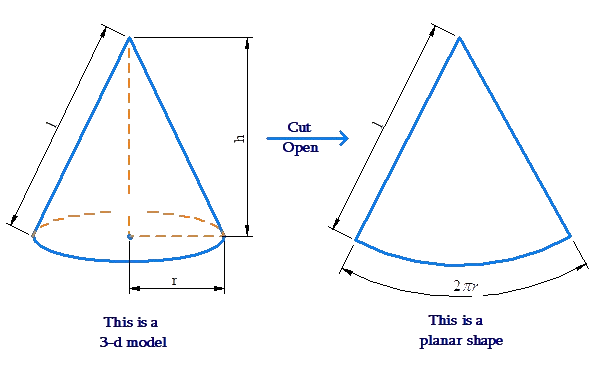# Surface Area of Cone

Go back to  'Mensuration-Solids'

The total surface area of a cone consists of two parts:

• The curved surface area

• The flat surface area (of the base)

The flat surface area is simply $$\pi {r^2}$$, since the base is circular. What is the curved surface area? To determine that, visualize what would happen if we cut open the cone and roll it out:We will obtain a circular sector. It would be a good idea to try this activity. Cut out a circular sector from a piece of paper. Then, fold / roll the paper so that the two straight edges align with each other, and you will get a cone.

Note that the radius R of the circular sector is the slant height of the cone. Denote the slant height by  $$l$$. Observe that $$l= \sqrt {{r^2} + {h^2}}$$. Also, the arc length L of the circular sector is the circumference of the cone’s base, which is $$2\pi r$$. Now, the area of the circular sector is

\begin{align}&A = \frac{1}{{2\pi }} \times \frac{L}{R} \times \pi {R^2}\,\,\,\left( {{\rm{why}}?} \right)\\&\,\,\,\,\, = \frac{{LR}}{2} = \frac{{\left( {2\pi r} \right)\left( l \right)}}{2}\\&\,\,\,\,\, = \pi rl\end{align}

This is also the curved surface area of the cone!

To summarize:

• Flat SA= $$\pi {r^2}$$

• Curved SA = $$\pi rl = \pi r\sqrt {{r^2} + {h^2}}$$

• Total SA = $$\pi {r^2} + \pi rl = \pi r\left( {r + l} \right)$$

## What is the formula for cones?

• The following are the 3 main formulas for a cone: Flat Surface Area= πr^2, Curved Surface Area = πrl=πr√r^2+h^2 and Total Surface Area = πr^2+πrl=πr(r+l)

## Why is the volume of a cone 1/3rd of a cylinder?

• Since the volume of a cone with radius r and height h is 1/3 * πr^2 h, it is exactly 1/3^rd the volume of the smallest cylinder that can fit inside the cone.

## How to calculate the surface area of a cone?

• the Total Surface Area of a cone can be calculated using the formula: πr^2+πrl=πr(r+l)

## What is the formula for circumference?

• the Total Surface Area of a cone can be calculated using the formula: πr^2+πrl=πr(r+l)

## How to find the volume of a cone?

• If the cone’s base radius is r and height is h, its volume is given by: V=1/3 * πr^2 h
Mensuration and Solids
Mensuration and Solids
grade 10 | Questions Set 2
Mensuration and Solids
Mensuration and Solids
grade 10 | Questions Set 1